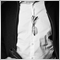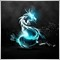# Python How to calculate the quantity by investment and leverage - page 22606

The documentation does state the formulas, I have posted the link.

You need to use this function, so that you get the factor, given by the specific symbol.

That's how the symbols information is incorporated into the accounts leverage.87

Well, the problem is that  margin_initial=0.0 for all the symbols while they have different one2606

Dragos Drimbe #:
Well, the problem is that  margin_initial=0.0 for all the symbols while they have different one

I will give a short snippet from my code, just to help you understand, it does not compile, nor is it complete....

```            #ifndef __MQL4_COMPATIBILITY_CODE__
if(!(::SymbolInfoMarginRate(symbol, ((bShort) ? ORDER_TYPE_SELL : ORDER_TYPE_BUY), margin_rate, maintenance_rate)))
{ return(NULL); }
#endif
}

// Select calculation type

switch(calc_mode)
{
case SYMBOL_CALC_MODE_FOREX:                    margin_rate         = (margin_maintenance) && (maintenance_rate > NULL) ? maintenance_rate : margin_rate;
symbol_leverage     = MathMax((int)((margin_rate > 1.0) ? (account_leverage / margin_rate) : account_leverage), 1);
return(((vol_size * contract_size) / account_leverage) * margin_rate);
```2606

Dragos Drimbe #:
Well, the problem is that  margin_initial=0.0 for all the symbols while they have different one

Margin Initial is only used for following calculation modes:

```SYMBOL_CALC_MODE_FUTURES
SYMBOL_CALC_MODE_EXCH_FUTURES
SYMBOL_CALC_MODE_EXCH_FUTURES_FORTS
```87

Margin maintanance is also 0 for all of them so I cant take your code and work on it, the only thing is order_calc_margin function but I have no idea how to use it2606

Dragos Drimbe #:
Margin maintanance is also 0 for all of them so I cant take your code and work on it, the only thing is order_calc_margin function but I have no idea how to use it

So why are you still using values, that are not applicable, obviously?

You need to apply some brains to the code I posted. - You are looking for margin_rate, as can be seen by my code.

`symbol_leverage     = MathMax((int)((margin_rate > 1.0) ? (account_leverage / margin_rate) : account_leverage), 1);`

Is it so, that nobody here understands the code, I've posted? - Or is it in fact you need spoon feeding the solution?

It is very hard to determin, if someone is simply not applying their brains, or are in fact not capable of reading the code.... - How am I supposed to tell the difference?

EDIT:

BTW, margin_maintenance in my code is a boolean operator, which determines, if you are querying for maintenance margin or for current margin rate...

Maybe you should first get familliar with margin and how it works, as it seems, lots of people have trouble with understanding how it works. But, if anyone is serious about their foregoing in trading, this should be basics, that need to be understood. - Thats almost like if you cannot read or write, but want to be an author... - Then first learn how to read and write, I would suggest, at least.87

Sry but i dont think I am advanced in programming. Is a hobby and I have little experience with complex things.

I have tryied your example and it give the right leverage only for one symbol of 5 tested and only if I devide the result bu 100

Leverage for each symbol

Rafael Sierra Clemente, 2021.10.31 19:48

I've calculated symbol leverage in this way:

```int CalculateSymbolLeverage(const string symbol)
{

int leverage=-1;
double margin=0.0;
double lots=1.0;

{
double lotValue = tickValue * SymbolInfoDouble(symbol,SYMBOL_ASK) / tickSize;
leverage=(int)MathRound(lotValue/margin);
}

return leverage;
}```
Regards

I have tried this code modified for python but it also give wrong values for a lot of symbols for example it gives 500 for symbols with 100 and everybody say is the tick value wrong. Now I presume I modified right. like I use ordin_calc_margin for margin value2606

Dragos Drimbe #:

Sry but i dont think I am advanced in programming. Is a hobby and I have little experience with complex things.

I have tryied your example and it give the right leverage only for one symbol of 5 tested and only if I devide the result bu 100

I have tried this code modified for python but it also give wrong values for a lot of symbols for example it gives 500 for symbols with 100 and everybody say is the tick value wrong. Now I presume I modified right. like I use ordin_calc_margin for margin value

Could you please share in PM the broker you are using, I want to see if my code calculates correctly, before giving you further advice.

PM because broker related details are not allowed on the forum.87

Sure I have send you a friend request as you have messages enabled only from friends